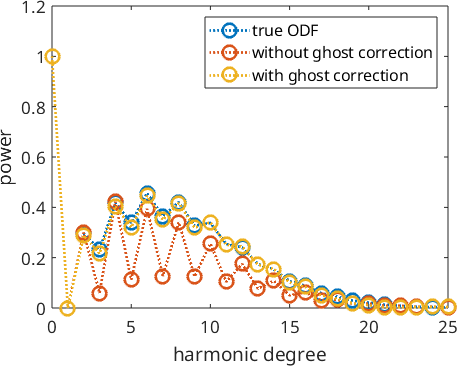Ghost Effect Analysis edit page

A general problem in estimating an ODF from pole figure data is the fact that the odd order Fourier coefficients of the ODF are not present anymore in the pole figure data and therefore it is difficult to estimate them. Artifacts in the estimated ODF that are due to underestimated odd order Fourier coefficients are called ghost effects. It is known that for sharp textures the ghost effect is relatively small due to the strict non-negativity condition. For weak textures, however, the ghost effect might be remarkable. For those cases, MTEX provides the option ghost_correction which tries to determine the uniform portion of the unknown ODF and to transform the unknown weak ODF into a sharp ODF by substracting this uniform portion. This is almost the approach Matthies proposed in his book (He called the uniform portion phon). In this section, we are going to demonstrate the power of ghost correction at a simple, synthetic example.

## Construct Model ODF

A unimodal ODF with a high uniform portion.

## Simulate pole figures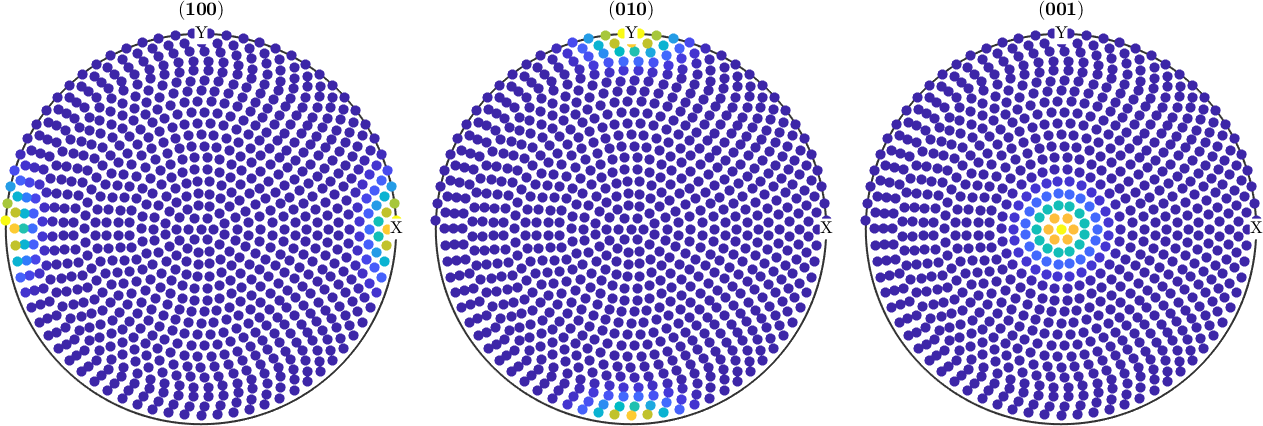## ODF Estimation

without ghost correction:

with ghost correction:

## Compare RP Errors

without ghost correction:

with ghost correction:

## Compare Reconstruction Errors

without ghost correction:

with ghost correction:

## Plot the ODFs

without ghost correction: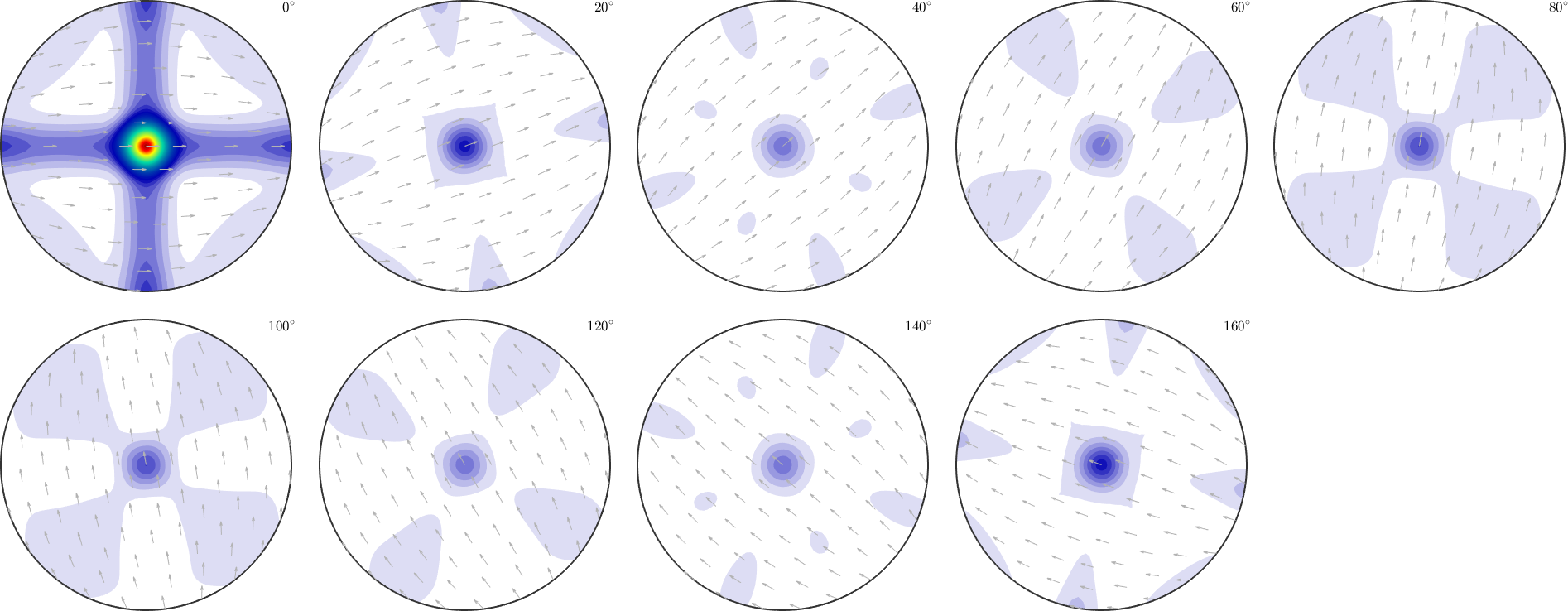with ghost correction: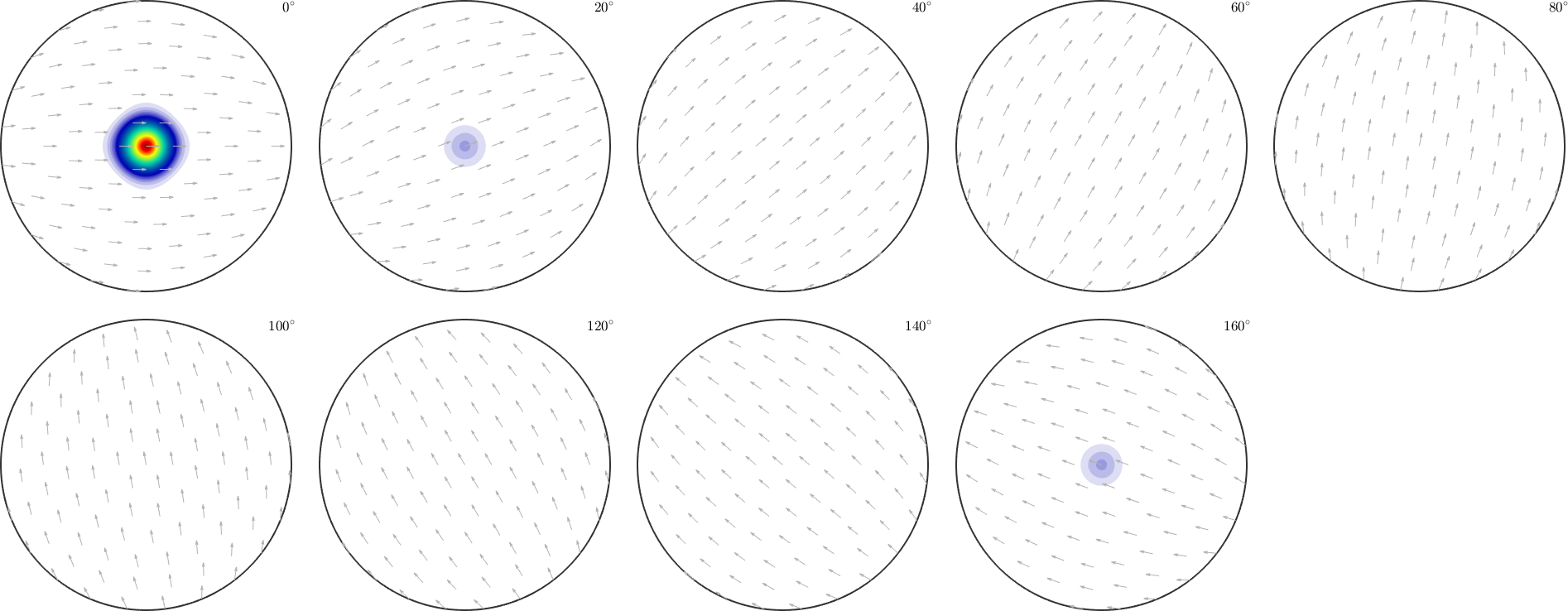radial plot of the true ODF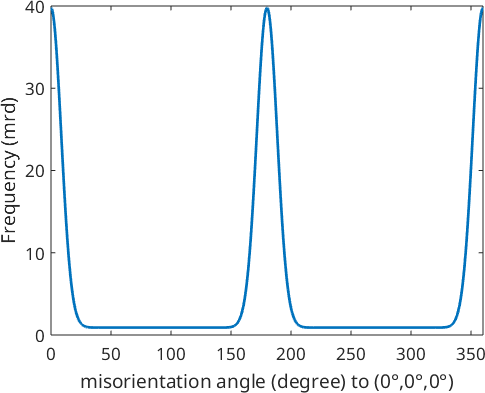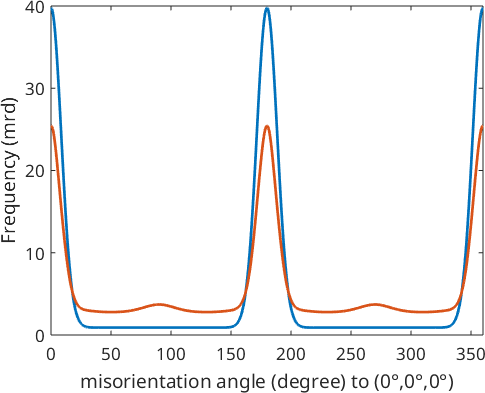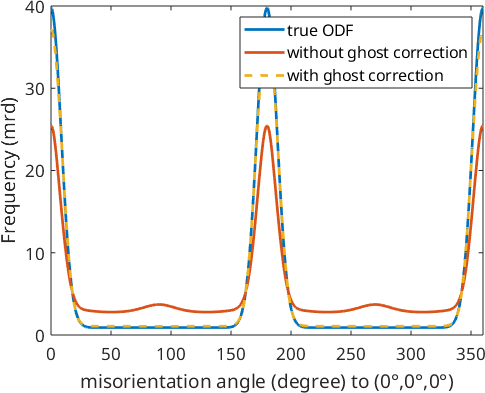## Calculate Fourier coefficients

Next, we want to analyze the fit of the Fourier coefficients of the reconstructed ODFs. To this end, we first compute Fourier representations for each ODF

## Calculate Reconstruction Errors from Fourier Coefficients

without ghost correction:

with ghost correction:

## Plot Fourier Coefficients

Plotting the Fourier coefficients of the recalculated ODFs shows that the Fourier coefficients without ghost correction oscillates much more than the Fourier coefficients with ghost correction

true ODF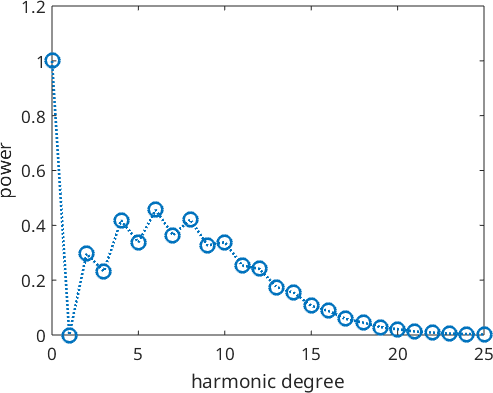keep plotting windows and add next plots

Without ghost correction: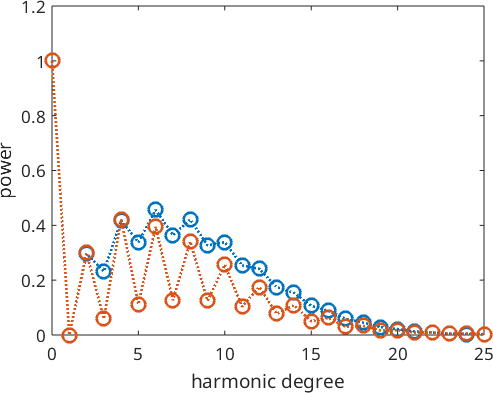with ghost correction